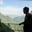Related Tags

java
communitycreator

# How to use Math.hypot() in Java?AKASH BAJWA

The hypot() method is a static method of the java.lang.Math library. It takes two numbers and returns the square root of $x^{2}$ + $y^{2}$ without any intermediate underflow or overflow.

There are special cases regarding this function:

• The result will be positiveinfinity if any of the parameters are infinite.

• The result will be NaN if any of the parameters are NaN and none of them are infinite.

### Syntax

public static double hypot(double x, double y)


### Parameters

This method will take two numbers of primitive data type double:

• x contains the first argument.
• y contains the second argument.

### Return value

This function will return the answer of √($x^{2}$ + $y^{2}$) without any overflow or underflow of double type.

### Example

This code illustrates how to use the hypot() method to compute sqrt ($x^{2}$ + $y^{2}$).

• We initialized num1= 32176.2 and num2= -298.77, then called the function to perform the calculations.
• It returns 32177.5870.
import java.lang.*;
public class EdPresso {
public static void main(String[] args) {
//initializng the variables
double num1 = 32176.2;
double num2 = -298.77;
//calling the hypot method
//and printing the output
}
}

RELATED TAGS

java
communitycreator

CONTRIBUTORAKASH BAJWA
RELATED COURSES

View all Courses

Keep Exploring

Learn in-demand tech skills in half the time